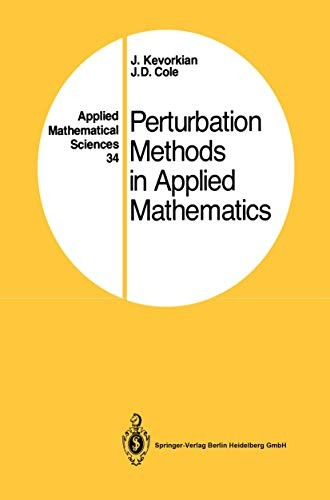# Perturbation Methods in Applied Mathematics by J. Kevorkian

Out of Stock
\$142.99
inc. GST
The basic ideas, however, are also applicable to integral equations, integrodifferential equations, and even to_difference equations.
Only 0 left

## Perturbation Methods in Applied Mathematics Summary

### Perturbation Methods in Applied Mathematics by J. Kevorkian

This book is a revised and updated version, including a substantial portion of new material, of J. D. Cole's text Perturbation Methods in Applied Mathe matics, Ginn-Blaisdell, 1968. We present the material at a level which assumes some familiarity with the basics of ordinary and partial differential equations. Some of the more advanced ideas are reviewed as needed; therefore this book can serve as a text in either an advanced undergraduate course or a graduate level course on the subject. The applied mathematician, attempting to understand or solve a physical problem, very often uses a perturbation procedure. In doing this, he usually draws on a backlog of experience gained from the solution of similar examples rather than on some general theory of perturbations. The aim of this book is to survey these perturbation methods, especially in connection with differ ential equations, in order to illustrate certain general features common to many examples. The basic ideas, however, are also applicable to integral equations, integrodifferential equations, and even to_difference equations. In essence, a perturbation procedure consists of constructing the solution for a problem involving a small parameter B, either in the differential equation or the boundary conditions or both, when the solution for the limiting case B = 0 is known. The main mathematical tool used is asymptotic expansion with respect to a suitable asymptotic sequence of functions of B.

### Why buy from World of BooksOur excellent value books literally don't cost the earthFree delivery in AustraliaEvery used book bought is one saved from landfill

1 Introduction.- 2 Limit Process Expansions Applied to Ordinary Differential Equations.- 3 Multiple-Variable Expansion Procedures.- 4 Applications to Partial Differential Equations.- 5 Examples from Fluid Mechanics.- Author Index.

GOR010226041
Perturbation Methods in Applied Mathematics by J. Kevorkian
J. Kevorkian
Applied Mathematical Sciences